Counting with Partitions

Peter Luschny,  2009-02-20

Abstract. We show how to generate the partitions of an integer in a tree-like manner and define on top of that the partition-product of two arithmetic functions. This partition-product provides a compact framework for generalized Stirling triangles and covers more than 120 sequences described at the On-Line Encyclopedia of Integer Sequences. For the convenience of OEIS users we compile two reference-tables weaving these sequences into a conceptual net.

Content

• Partition Trees
• Generating Integer Partitions
• The Partition Product
• Generalized Stirling Triangles
• Generalized Bessel Triangles

Partition Trees

A partition of n is defined as a sequence of nonnegative integers a1≥ a2≥...≥am such that n = a1 + a2 + ... + am for some m.

Partition-tree of  <6>  <7>  <8>  <9>.

Partitions of an integer can be generated in a natural way as a binary tree. D. E. Knuth writes: "All partitions of n into m parts appear on level n - m in lexicographic order; preorder of the entire tree gives lexicographic order of the whole." TAOCP 4, fasc. 3, 7.2.1.4, exercise 10. One can add: "All partitions of n with largest part k appear on the left subtree of 'k11..1', (n-k times 1)." Knuth gives reference to a more general tree-generating algorithm by T. I. Fenner and G. Loizou, Comp. J. 23 (1980), 332-337.

So reading the levels from left to right and from top to bottom an order is induced on the partitions. For example the partitions of 9 are ordered as shown in the list below.

[1, 1, 1, 1, 1, 1, 1, 1, 1]
[2, 1, 1, 1, 1, 1, 1, 1]
[2, 2, 1, 1, 1, 1, 1]
[3, 1, 1, 1, 1, 1, 1]
[2, 2, 2, 1, 1, 1]
[3, 2, 1, 1, 1, 1]
[4, 1, 1, 1, 1, 1]
[2, 2, 2, 2, 1]
[3, 2, 2, 1, 1]
[3, 3, 1, 1, 1]
[4, 2, 1, 1, 1]
[5, 1, 1, 1, 1]
[3, 2, 2, 2]
[3, 3, 2, 1]
[4, 2, 2, 1]
[4, 3, 1, 1]
[5, 2, 1, 1]
[6, 1, 1, 1]
[3, 3, 3]
[4, 3, 2]
[4, 4, 1]
[5, 2, 2]
[5, 3, 1]
[6, 2, 1]
[7, 1, 1]
[5, 4]
[6, 3]
[7, 2]
[8, 1]


In the chapter Combinatorial Analysis by K. Goldberg, M. Newman and E. Haynsworth in the Handbook of Mathematical Functions, (ed. Abramowitz and Stegun), page 831, table 24.2, the partitions for n = 1..10 are listed. However, the root of the partition-tree is put there on the bottom and the parts are written in ascending order. Thus the 'partitions'  in the Handbook are not partitions according to our formal definition, which is the standard definition in use today.

The reason why Goldberg, Newman and Haynsworth used this ordering in the Handbook seems quite clear: It is the order by which partitions were listed for more than 200 years by of one of most popular algorithms for generating partitions: The algorithm of C. F. Hindenburg (Göttingen 1779). Therefore we call this order the Hindenburg order, although this is not a standard term.

To give an illustration we list the partitions of 6 in the Hindenburg order:

6-15-24-33-114-123-222-1113-1122-11112-111111
[Hindenburg order]

Reading this line as a string (part by part, not partition by partition) from the right hand side to the left hand side we get another ordering, which we call the 'reflected Hindenburg order'.

111111-21111-2211-3111-222-321-411-33-42-51-6
[reflected Hindenburg order]

This ordering displays the partitions in accordance to the definition as a nonincreasing sequence and, furthermore, is exactly the ordering generated by the tree algorithm mentioned above. This is the ordering we will use here in all what follows.

There is another way to look at the reflected Hindenburg order (and why this is the standard order of partitions). It is the n-th level of the Hasse diagram in Alfred Young's lattice. Thus the partial order of the partitions implied by the structure of the generating tree is consistent with the refinement order induced from the lattice of partitions of an n-element set.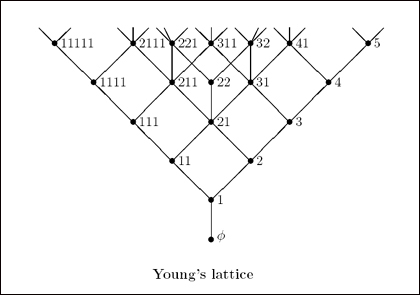Image by Prof. Richard Stanley. (Creative Commons License - MIT Open Courseware)

At the On-Line Encyclopedia of Integer Sequences (OEIS) the term 'Abramowitz-Stegun order' or even worse 'A-St order' is used to refer to the Hindenburg order: What a horrible misnomer!

Another caveat: Partition-based sequences start with offset 0, not 1. This is established by the basic sequences A000070 and A000041 at OEIS. '0' has a partition, the empty sequence.

Generating Integer Partitions

Below the tree-based generating algorithm for integer partitions is coded with Maple V. However, it does not use any special functions of Maple, only the predefined data-structure queue is used.The Partition Product

Now we can define an important combinatorial counting tool, the partition product of two functions f and g.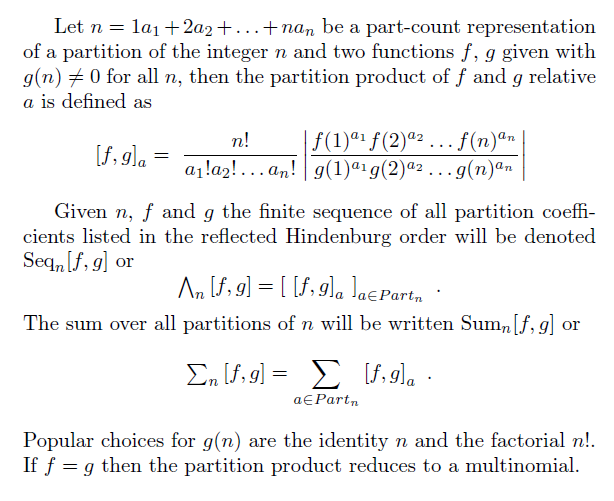The partition product is closely related to convolution polynomials, Jabotinsky matrices and Bell polynomials (where g(n) = n! and the f(n) are the 'variables'). See the paper "Convolution Polynomials" by Donald E. Knuth, page 8, available at arXiv.org. We implement the partition product as a Maple procedure, based on the partition generating algorithm.

However, let us first correct Maple's inadequate definition of the power function (compare D. E. Knuth, Two notes on notation).
pow := proc(n,k) if n=0 and k=0 then 1 else n^k fi end:

Now the list of partition products, i.e. Seqn[f, g], can be computed as: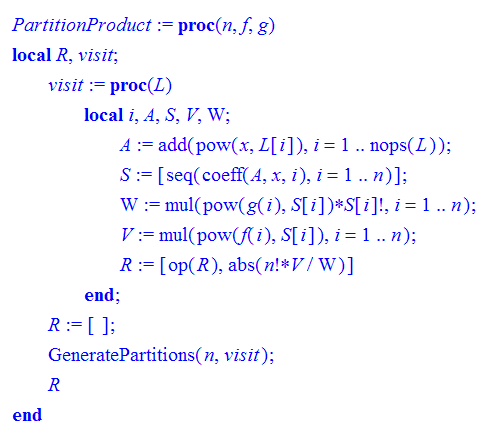Frequently we will focus on equivalence classes of partitions and it turns out that we gain much clarity if we look simultaneously at four equivalence relations from the very beginning. The first two are trivial: the identity which gives the fine-grained definition that every partition is a distinct partition and the coarse-grained definition that puts all partitions in the same pot. A third classification combines partitions with equal length and the fourth classification combines partitions whose biggest part are equal.

Counting with partitions means assigning a statistic to an equivalence class of partitions. In each case the partition product of the members of a class are summed up to a single value. To handle these cases uniformly we introduce a type statistic, where 'statistic' is in {"sum", "part", "len", "big"} and add this type as a parameter to the partition product which now has the signature PartitionProduct(n, f, g, statistic).  The correspondence is:

• coarse-grained -> "sum"
• fine-grained -> "part"
• equal length -> "len"
• equal biggest part -> "big"

In the tables below these statistics (which depend on the functions f and g of the partition product) are displayed in the captions of the tables like this:

 f(n) = .. ; g(n) = .. Sum All partitions ~ by length ~ by biggest part

To gain the extended functionality is easy. In Maple syntax it means that the concatenation operation R := R[op(L), U] (used in the "part" case) is replaced by R[nops(L)] := R[nops(L)] + U if the equivalence-type is 'length of partition' and by R[L] := R[L] + U if the equivalence-type is 'biggest part'.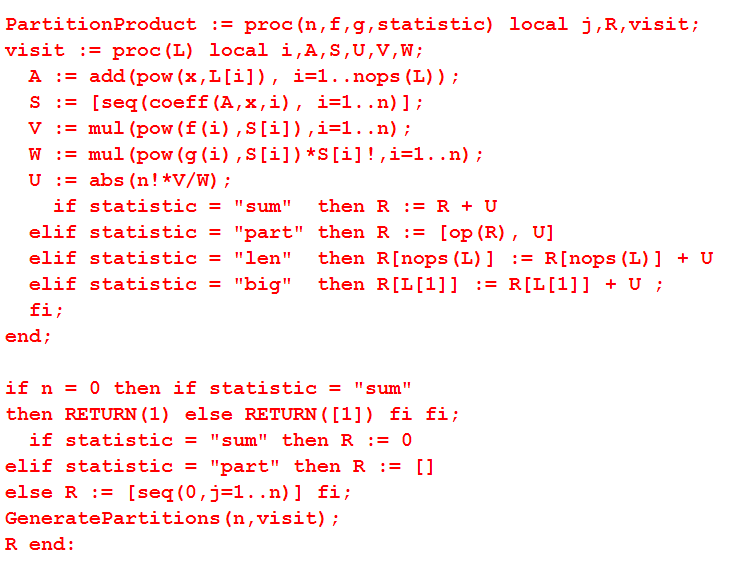To get an idea what this product produces we look first at three simple examples.

 f(n) = 1, g(n) = n! Sum: A000110 All partitions: A036040 ~ by length: A008277 ~ by biggest part: A080510 1    1    2 [1,1] [1,1] [1,1] 5 [1,3,1] [1,3,1] [1,3,1] 15 [1,6,3,4,1] [1,7,6,1] [1,9,4,1] 52 [1,10,15,10,10,5,1] [1,15,25,10,1] [1,25,20,5,1]

The partition product with 'part'-statistic gives the multinomial coefficients, with 'length'-statistic the Stirling numbers of the second kind and with 'biggest part'-statistic the set partitions of an n-set with maximum block-size k. The row sums are the Bell numbers.

 f(n) = n, g(n) = n! Sum: A000248 All partitions: A000000 ~ by length: A059297 ~ by biggest part: A000000 1    1    3 [1,2] [2,1] [1,2] 10 [1,6,3] [3,6,1] [1,6,3] 41 [1,12,12,12,4] [4,24,12,1] [1,24,12,4] 196 [1,20,60,30,60,20,5] [5,80,90,20,1] [1,80,90,20,5]

A000248 is the number of forests with n nodes and height at most 1, equivalently by a comment of R. Ferreol, the number of idempotent mappings f from a set of n elements into itself (i.e. satisfying f o f = f).  The length-statistic gives  the triangle of idempotent numbers (version 1).

The sequence A059297 is listed at OEIS in a slightly different way. Therefore we make up on defining the enumeration which we will use when we flatten the triangles:

 T(0,0) T(1,1) T(2,1), T(2,2) T(3,1), T(3,2), T(3,3) ...   ...   ...   ...

mapped to T(0,0), T(1,1), T(2,1), T(2,2), T(3,1), T(3,2), T(3,3),... Strictly speaking the enumeration at OEIS T(0,0),T(1,0),T(1,1),T(2,0),T(2,1),T(2,2), T(3,0),T(3,1),T(3,2),T(3,3),.. is more accurate. But many partition-based triangles at OEIS are based on the (1,1)-offset, so our enumeration fits better in with the actual OEIS-practice.

Our third example is

 f(n) = (n-1)!, g(n) = n! Sum: A000142 All partitions: A102189 ~ by length: A130534 ~ by biggest part: A126074 1    1    2 [1,1] [1,1] [1,1] 6 [1,3,2] [2,3,1] [1,3,2] 24 [1,6,3,8,6] [6,11,6,1] [1,9,8,6] 120 [1,10,15,20,20,30,24] [24,50,35,10,1] [1,25,40,30,24]

'All partitions' are counted by the multinomial numbers, which, classified by length, give the unsigned Stirling numbers of the first kind and classified by biggest part the permutations of n elements with longest cycle length k. The row sums are the factorial numbers.

Generalized Stirling Triangles

We will base our further exposition on a single function, the generalized rising factorial defined as:Our first application of the partition-product is a generalization of the Stirling numbers of the first kind, the Stirling1-Triangles(n,k), where n = 0, 1, 2, 3,... and k = ..., -3, -2, -1, 0, 1, 2, 3,... . A combinatorial interpretation of the generated combinatorial triangles below is given in part in the paper of Wolfdieter Lang, Combinatorial Interpretation of Generalized Stirling Numbers, preprint Oct 2008 and in Wolfdieter Lang, On generalizations of the Stirling number triangles, Journal of Integer Sequences, 3 (2000) Article 00.2.4. As a general introduction to the topic I recommend chapter 13, 'Partitions in Combinatorics', in George E. Andrews' The Theory of Partitions.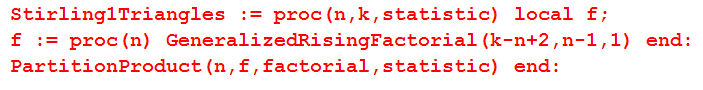Let's see what happens if we print these generalized Stirling_1 triangles:
for k from -6 to 5 do PrintTriangles(Stirling1Triangles, k, 5).

To open the frame in another window click: Stirling1-Triangles

The references to OEIS given are, with regard to the partition statistic, not fully correct. The partition sequences at OEIS are sorted by the Hindenburg order whereas we use the reflected Hindenburg order -- as we explained above. Therefore the rows of the triangles of the partition type (in the second column in the table above) are in reverse order compared to our results. To sum up: We have listed for -6 ≤ k ≤ 5 the partition-product of the function f (n) = GeneralizedRisingFactorial(k-n+2,n-1,1) with the factorial g(n) = n! in four flavors: 'all equal', 'all different', 'equal by length' and 'equal by biggest part'.

Let us look at some values of f as a function of n and k:

 1 1, 2 1, 3, 6 1, 4, 12, 24 1, 5, 20, 60, 120 1, 6, 30, 120, 360, 720

At OEIS we read: A008279 giving number of permutations of n things k at a time. Therefore the name generalized permutation coefficients for the triangles would also be appropriate.

This was quite successful. So let's try another one. This time we want to look at generalizations of the Stirling numbers of the second kind, Stirling2Triangles(n,k) (again see the Lang references for combinatorial interpretations).Let's see what happens this time:
for k from -6 to 5 do PrintTriangles(Stirling2Triangles, k, 5) od;

To open the frame in another window click: Stirling2-triangles. Another 60 OEIS-hits!

A particular case is noteworthy: for k = -2 the generalized Stirling triangles of the first and the second kind coincide. This is due to the fact that prod_{j=0..n-1}((k+1)*j-1) and prod_{j=0..n-2}(k-n+j+2) both have the absolute value n! if k = -2. This means that the signless Lah number can be seen as a generalized Stirling_1 triangle as well as a generalized Stirling_2 triangle. The same is true for the sequence with biggest-part statistic A157400.

We have listed for -6 ≤ k ≤ 5 the partition-product of the function f(n) = GeneralizedRisingFactorial(-1, n, k+1) with the factorial g(n) = n!. Let us look at some values of -f as a function of n and k:

 1 1, 2 1, 3, 21 1, 4, 36, 504 1, 5, 55, 935, 21505 1, 6, 78, 1560, 42120, 1432080

But now: surprise! I expected some well known sequence to appear, like the permutation coefficients in the case of the Stirling_1 triangles. However, this triangle is not at OEIS. What do these numbers basically count?

Summa summarum: We have looked at the partition-product of the generalized rising factorial for the parameter families

(n, k) → (k − n + 2, n − 1, 1)  and  (n, k) → (−1, n, k + 1)

with the factorial function n! and simultaneously looked at the general case and the cases induced by the equivalence relations 'length of partition' and 'biggest part of partition'. It turned out that this conceptual framework comprises more than 120 entries in the On-Line Encyclopedia of Integer Sequences.

Generalized Bessel Triangles

The Bessel numbers appear in many different situations. Thus it is not surprising to find them described about 10 times in OEIS (search for A144299.) Most entries look like this one:
 1 1, 1 1, 3, 0 1, 6, 3, 0 1, 10, 15, 0, 0 1, 15, 45, 15, 0, 0 1, 21, 105, 105, 0, 0, 0

The variants are rather dull: Some have the zeros in front, some abstain from enumerating the zeros and so on. All have one thing in common: the block of nonzero numbers is separated from the block of zeros. So on Dec 07, 2008 the editor of OEIS wrote: "... this entry is the last of the versions of the underlying triangle to be added to the OEIS ..." (A144331). What a pity: Here is a new variant which is really different ;-) Take the partition-product of the rising factorial (n, k) -> (k-n+2, n-1, 1) (the same as in the Stirling1 case above) but this time with the identity, and evaluate at k = 1.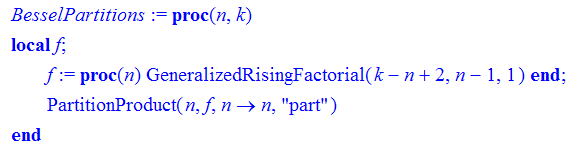Bessel numbers as BesselPartitions(n, 1).

   [1, 1] [1, 3, 0] [1, 6, 3, 0, 0] [1, 10, 15, 0, 0, 0, 0] [1, 15, 45, 0, 15, 0, 0, 0, 0, 0, 0] [1, 21, 105, 0, 105, 0, 0, 0, 0, 0, 0, 0, 0, 0, 0] [1, 28, 210, 0, 420, 0, 0, 105, 0, 0, 0, 0, 0, 0, 0, 0, 0, 0, 0, 0, 0, 0]

We can put this finding in a little formula. The row sums are the number of involutions In (self-inverse permutations) on n letters. Thus with the notation defined above we have

 In = Sumn[ (3-n)n-1, id ].

So perhaps the sequences In,k = Sumn[ (k-n+2)n-1, id ] should also be saved to Sloan's database?

We close with a table of generalized Bessel triangles.

To open the frame in another window click: Bessel-triangles